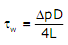# The partial differential equation is a

1.  Linear equation of order 2

2.  Non-linear equation of order 1

3.  Linear equation of order 1

4.  Non-linear equation of order 2

4

Non-linear equation of order 2

Explanation :
No Explanation available for this question

# The eigen values of symmetric matrix are all

1.  Complex with non-zero positive imaginary part

2.  Complex with non-zero negative imaginary part

3.  Real

4.  Pure imaginary

4

Real

Explanation :
No Explanation available for this question

# Match the CORRECT pairs Numerical Integration Scheme Order of Fitting Polynomial P. Simpson’s 3/8 Rule 1. First Q. Trapezoidal Rule 2. Second R. Simpson’s 1/3 Rule 3. Third

1.  P-2; Q-1; R-3

2.  P-3; Q-2; R-1

3.  P-1; Q-2; R-3

4.  P-3; Q-1; R-2

4

P-3; Q-1; R-2

Explanation :
No Explanation available for this question

# A rod of length L having uniform cross-sectional area A is subjected to a tensile  force P as shown in the figure below. If the Young’s modulus of the material varies linearly from E1 and E2 along the length of the rod, the normal stress  developed at the section-SS is

1.  P/A

2.  P(E1-E2)/A(E1+E2)

3.  PE2/AE1

4.  PE1/AE2

4

P/A

Explanation :
No Explanation available for this question

# The threaded bolts A and B of same material and length are subjected to identical  tensile load. If the elastic strain energy stored in bolt A is 4 times that of the bolt B and the mean diameter of bolt A is 12mm, the mean diameter of bolt B in mm is

1.  16

2.  24

3.  36

4.  48

4

24

Explanation :
No Explanation available for this question

# A  link  OB  is  rotating  with  a  constant  angular  velocity  of  2  rad/s  in  counter clockwise direction and a block  is  sliding  radially outward on  it with an uniform velocity  of  0.75 m/s with  respect  to  the  rod,  as  shown  in  the  figure  below.  If  OA = 1m, the magnitude of the absolute acceleration of the block at location A in m/s2 is:

1.  3

2.  4

3.  5

4.  6

4

5

Explanation :
No Explanation available for this question

# For steady,  fully developed  flow  inside a straight pipe of diameter D, neglecting gravity effects, the pressure drop  p   over a length L and the wall shear stress tw are related by

1.

2.

3.

4.

4Explanation :
No Explanation available for this question

# The pressure, dry bulb  temperature, and  relative humidity of air  in a  room are 1bar,  30ºC  and  70%  respectively.  If  the  saturated  steam  pressure  at  30ºC  is 4.25kPa, the specific humidity of the room air in kg water vapour / kg dry air is

1.  0.0083

2.  0.0101

3.  0.0191

4.  0.0232

4

0.0191

Explanation :
No Explanation available for this question

# Consider one-dimensional steady state heat conduction, without heat generation, in  a  plane  wall;  with  boundary  conditions  as  shown  in  the  figure  below.  The conductivity  of  the  wall  is  given  by K=K0+bT;  where K0  and b  are  positive constants and T is temperature As x increases, the temperature gradient  (dT / dx  ) will

1.  Remains constant

2.  Be zero

3.  Increase

4.  Decrease

4

Decrease

Explanation :
No Explanation available for this question

# In a rolling process, the state of stress of the material undergoing deformation is

1.  Pure compression

2.  Pure shear

3.  Compression and shear

4.  Tension and shear

4